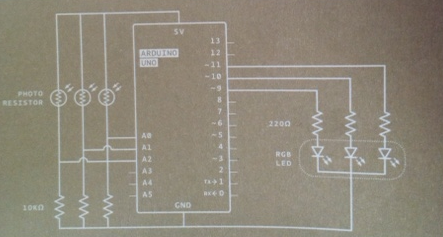# Understanding Project 4 circuit design

Hi everyone. I am trying to get a better understanding of the circuit in project 4. In particular, the part of the design I do not understand is the 10 kilo-ohm resistors which are plugged into the ground. I'm trying to visualize how the electricity is running through this circuit but I just don't understand those resistors. I feel very confident in my understanding of the circuits in the previous projects(where I feel I would be able to replicate the project without referencing the book), but I do not feel that way about this part of project 4.

Thanks for any helpCan you post the schematic? I've not seen the book you are talking about.Sorry about the quality, this is about as best I could do... here is a video of what the finished product looks like

Analog voltage= ~5V*10000/(photoresistor + 10000)
and
Analog Reading = ~Analog/5 * 1023

Without the 10K, the photoresistor just becomes a variable value pullup resistor that would read as 5V/1023 all the time.

Sorry, I'm a complete beginner with electronics and I don't really understand. This is the first time in the book I'm seeing one of these dividers. I think I understand the 5V*10000... 5 volts from the board, 10k from the resistor because Ohms law says volt s= current * resistence, but why are we dividing by (photoresistor + 10000)?
Again, I apologize if these questions seems silly, I've literally never worked on any sorts of electronics before. I am a programmer and my only experience with circuits are of the logical variety.

Ok, so V=IR, and V/R = I. Ohms Law.
There will a current flowing thru both resistors.
V/(photoresistor + 10000) = Itotal
The voltage across each resistor is then
V1= Itotal * photoresistor,
Vout = Itotal * 10000,
Vin = V1 +Vout
Put it all together, do some algebra, and you get
Vout = Vin * R2/(R1 +R2)
(and Vin = V1 + Vout, or Vin - Vout = V1)
R1 is the resistor between Vin and Vout (photoresistor), and R2 is the resistor between Vout and Gnd (10000).

Oh, I get it now! Thank you so much, that was very helpful!